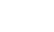# Chauvenet’s Criterion

Statistics Definitions > Chauvenet’s Criterion

## What is Chauvenet’s Criterion?

Chauvenet’s criterion is a way to identify outliers. The method works by creating an acceptable band of data around the mean, specifying any values that fall outside that band should be eliminated. The formula to calculate the band is:Where n is the sample size.
Normal distribution probabilities can be used to relate the formula to a maximum deviation from the mean:
τ = |Xi – x̄|/ s. This formula is a little more intuitive to use.

• The procedure should only be run once on a data set. Some authors advocate two runs; any outliers that are revealed on the second run are called shielded outliers. Note: other authors say never to eliminate outliers, so this is a judgment call based on your data.
• The procedure assumes your data is normally distributed.

## Running Chauvenet’s

You’ll probably want to perform these calculations in a spreadsheet, because the calculations can become numerous if you have multiple potential outliers (see Step 3).

For the following set of numbers, identify any outliers: 47, 50, 53, 55, 55, 56, 57, 57,58, 58, 58, 58, 60, 60, 60, 61, 61, 61, 61, 61, 61, 62, 62, 62, 63, 63, 64, 67, 68, 72.

Step 1: Calculate the sample mean, x̄. The sample mean is 59.7.

Step 2: Find the sample standard deviation, s. The sample standard deviation is 4.998.

Step 3: Use the following formula to find the standardized deviation from the mean for all suspected outliers, data values “i.”
τ = |Xi – x̄|/ s
We have thirty items in the set, so you’ll want to run the formula twenty times (one for each data point, i). For sake of brevity, I’m just going to show two of the data point calculations:
47: |47 – 59.7| / 4.998 = 2.541
67: |67 – 59.7| / 4.998 = 1.461

Step 4: Compare the values you got in Step 4 with a table of Chauvenet’s criterion values (see next section) to see if you can reject each data point. For n=30, the table tells us the band should end at 2.394 standard deviations.
47 is 2.541 standard deviations and is higher than 2.394, so should be eliminated. As 67 is under this figure, that data point stays.

If you’ve identified an abnormal amount of outliers, you might want to widen the band to include a certain percentage of data points. The empirical rule tells us that 95% of data lies within two standard deviations from the mean. Therefore a rule of thumb says that you should eliminate a maximum of 5% of your data points.

## Chauvenet’s Criterion Table

n τ
3 1.383
4 1.534
5 1.645
6 1.732
7 1.803
8 1.863
9 1.915
10 1.960
11 2.000
12 2.037
13 2.070
14 2.100
15 2.128
16 2.154
17 2.178
18 2.200
19 2.222
20 2.241
21 2.260
22 2.278
23 2.295
24 2.311
25 2.326
26 2.341
27 2.355
28 2.369
29 2.382
30 2.394
31 2.406
32 2.418
33 2.429
34 2.440
35 2.450
36 2.460
37 2.470
38 2.479
39 2.489
40 2.498
50 2.576
100 2.807
500 3.291
1000 3.481
CITE THIS AS:
Stephanie Glen. "Chauvenet’s Criterion" From StatisticsHowTo.com: Elementary Statistics for the rest of us! https://www.statisticshowto.com/chauvenets-criterion/
---------------------------------------------------------------------------Need help with a homework or test question? With Chegg Study, you can get step-by-step solutions to your questions from an expert in the field. Your first 30 minutes with a Chegg tutor is free!